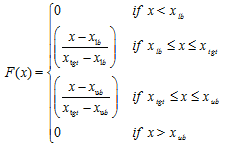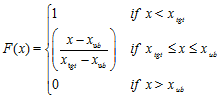Include Top

# Optimization Methods

DiscoverSim optimization features include:

• Dividing Rectangles (DIRECT) Global Optimization
• Genetic Algorithm (GA) Global Optimization
• Sequential Quadratic Programming (SQP) for Fast Local Optimization
• Powerful hybrid of the above 3 methods
• Stochastic Optimization – minimize dpm or maximize Ppk
• Multiple Response Optimization using Desirability, Weighted Sum or Deviation from Target
• Optimization with Input Controls
• Specify linear and nonlinear Constraints

Components of Optimization

Output: The output will be a function of the model equation, intermediate cell calculations, as well as input distributions and input controls.

Input Control: The permissible range for the control is specified, and the control, which unlike an input distribution, has no statistical variation. Think of this as a control knob like temperature. This is also known as a “Decision Variable”. An input control can be referenced by an input distribution parameter, constraint and/or an output function. It is possible to have a model that consists solely of controls with no input distributions. (In this case, the optimization is deterministic, so the number of replications, n, should be set to 1.) An input control can be continuous or discrete integer.

Constraint: A constraint can only be applied to an Input Control and cannot reference an Input Distribution or Output Response. A constraint cannot be a part of the model equation (i.e., an output cannot reference a constraint). Constraints can be simple linear or complex nonlinear.

Each constraint will contain a function of Input Controls on the Left Hand Side (LHS), and a constant on the Right Hand Side (RHS). Optimization will usually occur more rapidly if the initial values of the parameters and/or controls satisfy the constraint. In addition, optimization is much harder with equality constraints than with inequality constraints. Constraints are managed automatically as part of the SQP process; for DIRECT and GA, a penalty function is specified for each constraint - if the constraint is violated, the penalty should be increased. The default penalty function is 100.

Specifying the Optimization Objective Function

The following table gives the options available to specify an optimization objective function in the Run Optimization dialog:

 Optimization Goal: Minimize Maximize Multiple Output Metric: Weighted Sum Deviation from Target Weighted Sum Desirability Statistic: Mean Median1st quartile3rd quartileMinimumMaximum Standard DeviationVarianceMean Squared Error SkewnessKurtosisRangeIQR (75-25)Span (95-5) Actual DPM (defects per million)Calculated DPM (defects per million assuming normal distribution) Mean Mean Median1st quartile3rd quartileMinimumMaximum Standard DeviationVarianceMean Squared Error SkewnessKurtosisRangeIQR (75-25)Span (95-5) PpPpuPplPpkCpm%Pp (Percentile Pp) %Ppu (Percentile Ppu)%Ppl (Percentile Ppl)%Ppk (Percentile Ppk) Mean

Notes on Optimization:

1. Minimize/Maximize Weighted Sum: minimize or maximize the weighted sum of statistic. Weights are specified for each output in the Output Response dialog. For example, if the selected statistic was the Mean, and there were two outputs, the objective function would be:

2. Weight1Mean1 + Weight2Mean2

If there is a single output in the model, this simplifies to minimize or maximize the statistic value. Note that the Output Goal specified in the Output Response dialog is not used here. It is only used in Maximize Desirability.

3. Minimize Deviation from Target: minimize the square root of weighted sum of deviations squared. A target must be specified for each output in the Output Response dialog. The only statistic available for this option is the mean. This is also known as the Taguchi or Quadratic Loss Function. If there were two outputs, the objective function would be:

4. SQRT( Weight1(Mean1 – Target1)2 + Weight2(Mean2 – Target2)2).

5. Maximize Desirability: Maximize the weighted linear sum of the Derringer and Suich desirability measure (Derringer and Suich,1980). Each output must specify:

• Weight (default = 1). This is also referred to as “Importance”. (Note, another factor, the desirability shape is sometimes called “weight”. In DiscoverSim, the desirability shape factor is fixed at 1.)

• Output Goal (Target, Maximize or Minimize) – this is specific to an output. For example, if Output 1 is production rate, the goal would be set to maximize, and Output 2, cost, would have a minimize goal. However the specified overall Optimization Goal is to maximize desirability.

• If the output goal is Target, then LSL, Target, and USL are required. LSL and USL are the lower and upper specification limits used for process capability and dpm calculations, but are also used as the lower and upper bounds for desirability. The objective function is:

•• If the output goal is Minimize, then Target and USL are required. The objective function is:

•The only statistic available for the desirability option is the mean.

6. If the number of replications is set to n =1 for deterministic optimization, the statistic used is the mean, regardless of what has been selected.

7. Stochastic optimization requires a fixed seed in order to avoid “chatter” that would result in inconsistent comparisons. If the Seed is set to Clock, the initial seed value will be obtained from the system clock and then kept fixed throughout the optimization.

# Web Demos

Our CTO and Co-Founder, John Noguera, regularly hosts free Web Demos featuring SigmaXL and DiscoverSim